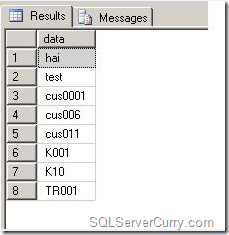### Sort Alphanumeric Data in SQL Server

This post shows how to sort alphanumeric data in SQL Server. You need to use a different approach to get an alphanumeric sort.

Consider the following code

Suppose you want to sort the string based on the alphabets first, followed by a number and if the string does not have any numeric value, then that string should come first in the sort

The following query will sort as explained above

Here’s the same query to try at your end

select data from @t
order by case
when data like '%[0-9]%' then 1
else 0
end,
data

The logic is to see if there are numbers in the string. If the data has numbers, it will be assigned 1 otherwise 0. So the records with 0 will be sorted first, then followed by 1. At the end, the final result will be sorted by data, as shown below. Observe that the string that do not have a number in it appears first, while sorting.